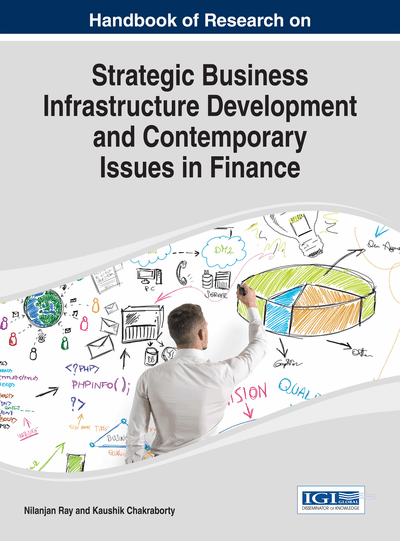# Risk-Based Selection of Portfolio: Heuristic Approach

Soma Panja, Dilip Roy
DOI: 10.4018/978-1-4666-5154-8.ch016
OnDemand:
(Individual Chapters)
Available
\$37.50
No Current Special Offers

## Abstract

This chapter examines the closeness between the optimum portfolio and portfolio selected by an investor who follows a heuristic approach. There may be basically two ways of arriving at an optimum portfolio – one by minimizing the risk and the other by maximizing the return. In this chapter, the authors propose to strike a balance between these two. The optimum portfolio has been obtained through a mathematical programming framework so as to minimize the portfolio risk subject to return constraint expressed in terms of coefficient of optimism (a), where a varies between 0 to 1. Simultaneously, the authors propose to develop four heuristic portfolios for the optimistic and pessimistic investors, risk planners, and random selectors. Given the optimum portfolio and a heuristic portfolio, City Block Distance has been calculated to measure the departure of the heuristic solution from the optimum solution. Based on daily security wise data of ten companies listed in Nifty for the years 2004 to 2008, the authors have obtained that when the value of a lies between 0 to 0.5, the pessimistic investor's decision is mostly closest to the optimum solution, and when the value of a is greater than 0.5, the optimistic investor's decision is mostly near to the optimum decision. Near the point a = 0.5, the random selectors and risk planners' solutions come closer to the optimum decision. This study may help the investors to take heuristic investment decision and, based on his/her value system, reach near to the optimum solution.
Chapter Preview
Top

## 1. Introduction

### 1.1. The Concerns

In asset allocation analysis one mostly uses the mean – variance approach for analyzing the tradeoff between risk and expected return. Since the seminal work of Markowitz (1952, 1959) the computational aspects of finding efficient portfolios have been a concern of the finance profession. In Markowitz portfolio theory, the portfolio of financial assets is characterized by a desired property, the ‘reward’, and something undesirable, the ‘risk’. Balancing financial aspects against statistical and, particularly, computational limitations, Markowitz identified reward and risk with the expectation and the variance of returns, respectively.

Since 1952, many works have been done to select an optimum portfolio. But in most of the cases it is difficult to arrive at an optimal solution. The main problem of selection of optimum portfolio is to obtain correct and precise portfolio weights (the proportion of wealth invested in each individual asset). If weights can be precisely estimated, it can reduce unnecessary transaction costs and help to construct the optimum portfolio. From a theoretical point of view, one should optimize expected utility for which several techniques are there to optimize the portfolio of which mean-variance approach can be an option. At a given point of time and a given set of conditions, heuristic solution may give reasonable result, and heuristically one can reach to the optimum or near optimum solution.

The portfolio optimization problem is mainly concerned with selecting the optimal investment strategy of an investor without considering the value system. In other words, the investor looks for an optimal decision which eventually is number of shares of which security the investor should purchase to maximize their expected utility. If the investor knows the securities that may give them maximum expected return or minimum expected risk, it is easy for them to take optimal decision. But in real world it is difficult to find out those securities due to presence of efficient market. In this empirical study we have tried to find out a heuristic solution with four different value systems which can help an investor to take decision and ascertain the portfolio based on the nearness to the optimum decision.

Roy, Mitra, and Chowdhury (2010) have proposed heuristic procedures to compute securities weights based on the investors’ propensity to take risk. They have chosen two extreme situations – risk taker and risk aversive investor. By constructing heuristic portfolios, they have shown the extent of closeness between the ideal portfolio constructed on the basis of optimization method and portfolio constructed on the basis of heuristic methods. In this work we tried to extend that work in terms of initial choice of the securities, construction of distance metric and value system of the investor.

In their empirical study, Roy, Mitra, and Chowdhury (2010) have considered big-cap companies of Nifty. As a result, it was more of a purposive selection. Random selection techniques for selection of companies were not considered in their study. Because of this non probabilistic selection, the conclusions drawn therein may not be extendable for the set of all securities. The next limitation arises out of a consideration of only two value systems covering pessimistic investors and optimistic investors.

## Key Terms in this Chapter

Risk: The probability that an actual return on an investment will be lower than the expected return.

Portfolio: A collection of assets held by an institution or a private individual.

## Complete Chapter List

Search this Book:
Reset